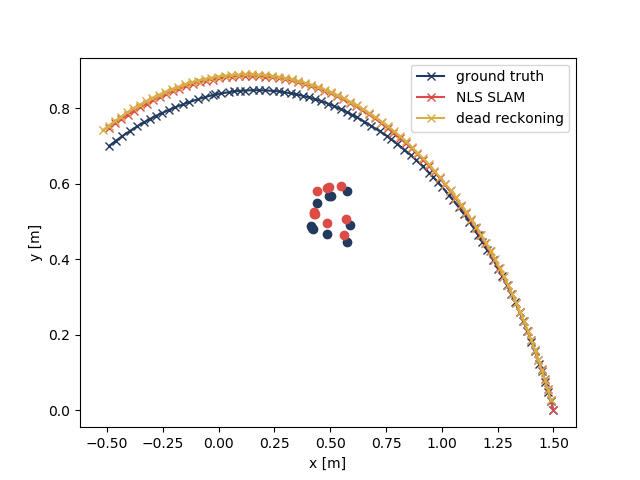16 Jul

#### Optimization tools for SLAM problems in Python

I wrote a 2D SLAM simulation on Python as a starting point. I implemented the nonlinear least squares (NLS) SLAM. To be specific, it is just to solve:

$$(\hat{s}_{1:n}, \hat{\lambda}) = {arg\,min}_{({s}_{1:n}, {\lambda})}\, \sum_{t=0}^{n-1} \| s_{t+1} - f(s_t, u_t) \|^2_{Q} + \sum_{t=1}^n \| o_t - h(s_t, \lambda) \|^2_{R}.$$

There are several optimization tools out there, including g2o and Ceres Solver. However, most of them are for C++. As I try to write everything in Python, I have to look for optimization tools in Python. The following is my journey.

### least_squares() in SciPy

As the NLS SLAM is a nonlinear least squares problem, this function is probably the first candidate everyone will arrive. It also supports the loss function $$\rho(\cdot)$$ to eliminate the outliers. However, this tool can only deal with single input, rather than the vector input with specified covariance. I discarded this tool in 10 min.

### curve_fit() in SciPy

Different from least_squares(), curve_fit() allows the user to specify the covariance, but not the loss function. After all, it seemed enough for a simple 2D SLAM example.

For my first few trials, the results from curve_fit() were not different from the dead-reckoning results, which I used as the initial estimates. One has to give the the Jacobian matrix for this optimization tool. That is, even though this tool claims it can estimate the Jacobian matrix numerically, it is not that powerful.

In fact, this is not the worst problem. When the dimension of the covariance matrix gets large, this tool suffers from severe numerical issues. For example, the inverse of a big covariance matrix is no longer positive definite due to the numerical rounding error. This method works only when the covariance is left default.

However, the limitation of this tool actually sheds light on the importance of general graph based optimization tools, like g2o.

### g2opy

g2o is a C++ framework for optimizing graph-based nonlinear error functions, and g2opy serves as a Python interface to access this tool. The main problem of g2opy is that it does not have well-written documents, and most of the examples are for computer vision rather than for robotics. The implementation of g2o should be straightforward for those who have solid programming background, but I did struggle for a little bit.

It finally works!